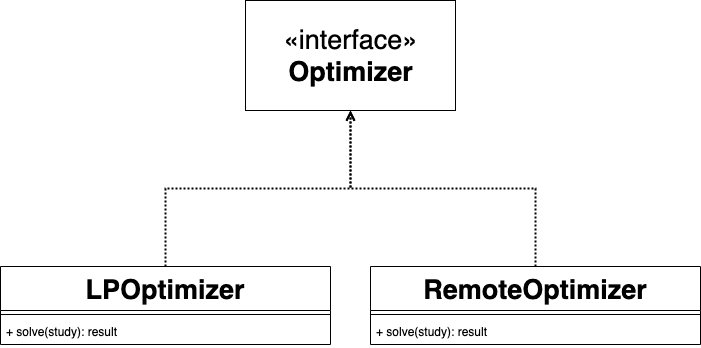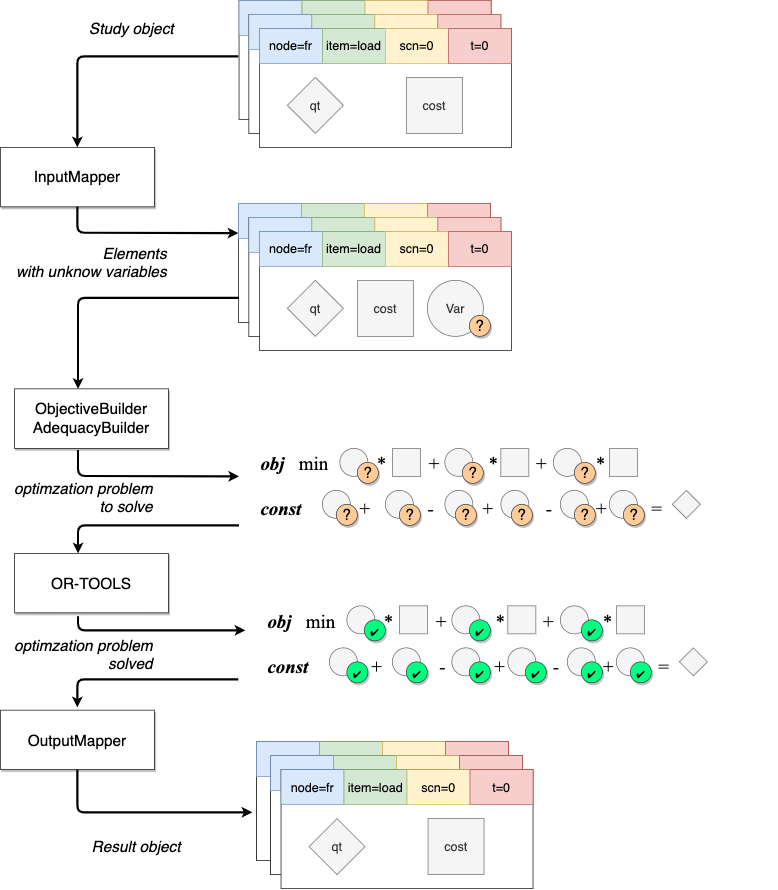# Optimizer¶

Optimizer is the heart of Hadar. Behind it, there are :

1. Input object called Study. Output object called Result. These two objects encapsulate all data needed to compute adequacy.

2. Many optimizers. User can chose which will solve study.

Therefore Optimizer is an abstract class build on Strategy pattern. User can select optimizer or create their own by implemented Optimizer.solve(study: Study) -> Result

Today, two optimizers are present LPOptimizer and RemoteOptimizer## RemoteOptimizer¶

Let’s start by the simplest. RemoteOptimizer is a client to hadar server. As you may know Hadar exist like a python library, but has also a tiny project to package hadar inside web server. You can find more details on this server in this repository.

Client implements Optimizer interface. Like that, to deploy compute on a data-center, only one line of code changes.

import hadar as hd
# Normal : optim = hd.LPOptimizer()
optim = hd.RemoteOptimizer(host='example.com')
res = optim.solve(study=study)


## LPOptimizer¶

LPOptimizer translate data into optimization problem. Hadar algorithms focus only on modeling problem and uses or-tools to solve problem.

To achieve modeling goal, LPOptimizer is designed to receive Study object, convert data into or-tools Variables. Then Variables are placed inside objective and constraint equations. Equations are solved by or-tools. Finally Variables are converted to Result object.

Analyze that in details.

### InputMapper¶

If you look in code, you will see three domains. One at hadar.optimizer.input, hadar.optimizer.output and another at hadar.optimizer.lp.domain . If you look carefully it seems the same Consumption , OutputConsumption in one hand, LPConsumption in other hand. The only change is a new attribute in LP* called variable . Variables are the parameters of the problem. It’s what or-tools has to find, i.e. power used for production, capacity used for border and lost of load for consumption.

Therefore, InputMapper roles are just to create new object with ortools Variables initialized, like we can see in this code snippet.

# hadar.optimizer.lp.mapper.InputMapper.get_var
cost=float(l.cost),
src=name,
quantity=l.quantity[scn, t],
variable=self.solver.NumVar(0, float(l.quantity[scn, t]),
'link on {} to {} at t={} for scn={}'.format(name, l.dest, t, scn)
)
)


### OutputMapper¶

At the end, OutputMapper does the reverse thing. LP* objects have computed Variables. We need to extract result found by or-tool to Result object.

Mapping of LPProduction and LPLink are straight forward. I propose you to look at LPConsumption code

self.nodes[name].consumptions[i].quantity[scn, t] =
vars.consumptions[i].quantity - vars.consumptions[i].variable.solution_value()


Line seems strange due to complex indexing. First we select good node name, then good consumption i, then good scenario scn and at the end good timestep t. Rewriting without index, this line means :

$Cons_{final} = Cons_{given} - Cons_{var}$

Keep in mind that $$Cons_{var}$$ is the lost of load. So we need to subtract it from initial consumption to get really consumption sustained.

### Modeler¶

Hadar has to build problem optimization. These algorithms are encapsulated inside two builders.

ObjectiveBuilder takes node by its method add_node. Then for all productions, consumptions, links, it adds $$variable * cost$$ into objective equation.

StorageBuilder build constraints for each storage element. Constraints care about a strict volume integrity (i.e. volume is the sum of last volume + input - output)

ConverterBuilder build ratio constraints between each inputs converter to output.

AdequacyBuilder is a bit more tricky. For each node, it will create a new adequacy constraint equation (c.f. Linear Model). Coefficients, here are 1 or -1 depending of inner power or outer power. Have you seen these line ?

self.constraints[(t, link.src)].SetCoefficient(link.variable, -1)  # Export from src


Hadar has to set power importation to dest node equation. But maybe this node is not yet setup and its constraint equation doesn’t exist yet. Therefore it has to store all constraint equations and all link capacities. And at the end build() is called, which will add importation terms into all adequacy constraints to finalize equations.

def build(self):
"""
Call when all node are added. Apply all import flow for each node.

:return:
"""
for (t, src, dest), var in self.importations.items():
self.constraints[(t, dest)].SetCoefficient(var, 1)


solve_batch method resolve study for one scenario. It iterates over node and time, calls InputMapper, then constructs problem with *Buidler, and asks or-tools to solve problem.

solve_lp applies the last iteration over scenarios and it’s the entry point for linear programming optimizer. After all scenarios are solved, results are mapped to Result object.#### Or-tools, multiprocessing & pickle nightmare¶

Scenarios are distributed over cores by mutliprocessing library. solve_batch is the compute method called by multiprocessing. Therefore all input data received by this method and output data returned must be serializable by pickle (used by multiprocessing). However, output has ortools Variable object which is not serializable.

Hadar doesn’t need complete Variable object. Indeed, it just want value solution found by or-tools. So we will help pickle by creating more simpler object, we carefully recreate same API solution_value() to be compliant with downstream code

class SerializableVariable(DTO):
def __init__(self, var: Variable):
self.val = var.solution_value()

def solution_value(self):
return self.val


Then specify clearly how to serialize object by implementing __reduce__ method

# hadar.optimizer.lp.domain.LPConsumption
def __reduce__(self):
"""
Help pickle to serialize object, specially variable object
:return: (constructor, values...)
"""
return self.__class__, (self.quantity, SerializableVariable(self.variable), self.cost, self.name)


It should work, but in fact not… I don’t know why, when multiprocessing want to serialize returned data, or-tools Variable are empty, and mutliprocessing failed. Whatever, we just need to handle serialization oneself

# hadar.optimizer.lp.solver._solve_batch
return pickle.dumps(variables)


## Study¶

code

Study is a API object I means it encapsulates all data needed to compute adequacy. It’s the glue between workflow (or any other preprocessing) and optimizer. Study has an hierarchical structure of 3 levels :

1. study level with set of networks and converter (Converter)

2. network level (InputNetwork) with set of nodes.

3. node level (InputNode) with set of consumptions, productions, storages and links elements.

4. element level (Consumption, Production, Storage, Link). According to element type, some attributes are numpy 2D matrix with shape(nb_scn, horizon)

Most important attribute could be quantity which represent quantity of power used in network. For link, is a transfert capacity. For production is a generation capacity. For consumption is a forced load to sustain.

### Fluent API Selector¶

User can construct Study step by step thanks to a Fluent API Selector

import hadar as hd

study = hd.Study(horizon=3)\
.network()\
.node('a')\
.consumption(cost=10 ** 6, quantity=[20, 20, 20], name='load')\
.production(cost=10, quantity=[30, 20, 10], name='prod')\
.node('b')\
.consumption(cost=10 ** 6, quantity=[20, 20, 20], name='load')\
.production(cost=10, quantity=[10, 20, 30], name='prod')\
.link(src='a', dest='b', quantity=[10, 10, 10], cost=2)\
.link(src='b', dest='a', quantity=[10, 10, 10], cost=2)\
.build()

optim = hd.LPOptimizer()
res = optim.solve(study)


In the case of optimizer, Fluent API Selector is represented by NetworkFluentAPISelector , and NodeFluentAPISelector classes. As you assume with above example, optimizer rules for API Selector are :

• API flow begin by network() and end by build()

• You can only downstream deeper step by step (i.e. network() then node(), then consumption() )

• But you can upstream as you want (i.e. go direcly from consumption() to network() or converter() )

To help user, quantity and cost fields are flexible:

• lists are converted to numpy array

• if user give a scalar, hadar extends to create (scenario, horizon) matrix size

• if user give (horizon, ) matrix or list, hadar copies N time scenario to make (scenario, horizon) matrix size

• if user give (scenario, 1) matrix or list, hadar copies N time timestep to make (scenario, horizon) matrix size

Study includes also check mechanism to be sure: node exist, consumption is unique, etc.

## Result¶

Result look like Study, it has the same hierarchical structure, same element, just different naming to respect Domain Driven Development . Indeed, Result is used as output computation, therefore we can’t reuse the same object. Result is the glue between optimizer and analyzer (or any else postprocessing).

Result shouldn’t be created by user. User will only read it. So, Result` has not fluent API to help construction.